Next: Level Raising and Lowering Up: An Example: Computing Previous: Source Code   Contents

## Future Problems

There remain many exciting problems to consider in the computation of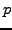-adic heights for elliptic curves. Below we outline a few:

Problem 6.2.4   Are there simplifications one could make to the above algorithm taking into account the fact that we're working with elliptic curves (e.g., using group structure, etc.)? Should we expect that the matrix of Frobenius be easier to compute in the case of genus 1 curves?

Problem 6.2.5   A question of John Tate: how does the cyclotomic-adic height pairing change for families of elliptic curves, e.g.,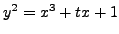? What about considering families with constant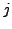-invariant? Non-constant-invariant? Curves with complex multiplication? Curves without complex multiplication?

Remark 6.2.6 (From Christian Wuthrich)   In my J. London Soc. article I computed some and proved that they are locally analytic. The height of a section can have zeroes as a rigid-adic analytic function. If the conjecture on the non-degeneracy of the heights is true, then the zeroes are not in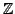but in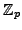. But I have no interpretation of what they could mean.

Problem 6.2.7   Extend the above algorithm to implement the computation of-adic anticyclotomic heights, using new ideas of Mazur in [MST06].

Problem 6.2.8 (From Christian Wuthrich.)   For computational reasons it would be interesting to also include the primes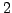and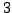. It should be possible to write a more complicated Kedlaya algorithm at least for. In shark I reprogrammed the original approximation algorithm (beware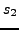is not exactly integral) forandso that I can compute-primary parts of Sha. Kato's theorem is not known to me for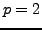.

Problem 6.2.9 (From Christian Wuthrich.)   There is a well-defined supersingular theory explained by Perrin-Riou. The Kedlaya algorithm can be used to compute the-adic heights also in this case. As I did it in shark. In shark there are also-adic heights for multiplicative primes using Mazur-Tate-Teitelbaum. Additive primes are a bit harder, one needs to pass to an extension over which they become semi-stable. So this calls for-adic height over arbitrary number fields.Next: Level Raising and Lowering Up: An Example: Computing Previous: Source Code   Contents
William Stein 2006-10-20